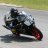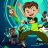VIX Fear & Greed Mean Reversion Indicator for ThinkorSwimBenTen

Staff
VIP
Warehouse
Similar to the previous Fear and Greed indicator, this one was designed for the \$VIX. The strategy generate buy and sell signals in ThinkorSwim using Bollinger Bands, Percent B (%B), and a few other parameters.

Calls

• go long VIX calls when %B(20,1.5) crosses > 0
• cover VIX calls when %B(20,1.5) crosses > 100

Puts
• go long VIX puts when %B(20,1.2) crosses < 100
• cover VIX puts when %B(20,2) crosses < 0VIX Fear and Greed Strategy

Rich (BB code):
#
# \$VIX Fear & Greed Mean Reversion Study (VIX_FGMR)
#
# This script adapted from posts from @kerberos007
#
# Want the latest version of this script?
# https://github.com/korygill/technical-analysis
#
# Use on thinkorswim and thinkscript
# author @korygill
#

script GetBollingerBandPercent
{
input price = close;
input upper = 2;
input lower = -2;
input averageType = AverageType.SIMPLE;
input displace = 0;
input length = 20;

def upperBand = BollingerBands(price, displace, length, lower, upper, averageType).UpperBand;
def lowerBand = BollingerBands(price, displace, length, lower, upper, averageType).LowerBand;

plot BBPercent = (price - lowerBand) / (upperBand - lowerBand) * 100;
}

declare lower;

input sym = "VIX";
def price = close(sym);
input averageType = AverageType.SIMPLE;
input displace = 0;
input length = 20;
input signalType = {default CALL, PUT};

plot PB20 = GetBollingerBandPercent(price, 2.0, -2.0);
plot PB15 = GetBollingerBandPercent(price, 1.5, -1.5);
plot PB12 = GetBollingerBandPercent(price, 1.2, -1.2);
plot PB10 = GetBollingerBandPercent(price, 1.0, -1.0);
#plot symPlot = price;

plot ZeroLine = 0;
plot HalfLine = 50;
plot UnitLine = 100;
ZeroLine.SetDefaultColor(Color.GREEN);
HalfLine.SetDefaultColor(GetColor(8));
UnitLine.SetDefaultColor(Color.RED);

def callput;
switch (signalType)
{
case CALL:
callput = 1;
default:
callput = 0;
}

# -----------------
Crosses(PB20, ZeroLine, CrossingDirection.BELOW) and callput == 0,
"--- (OS - Cover Puts) ---", Color.MAGENTA, curve.SHORT_DASH
);

Crosses(PB12, UnitLine, CrossingDirection.BELOW) and callput == 0,
"--- (OB - Buy Puts) ---", Color.YELLOW, curve.SHORT_DASH
);

# -----------------
Crosses(PB15, ZeroLine, CrossingDirection.ABOVE) and callput == 1,
"+++ OS - Buy Calls +++", Color.GREEN, curve.SHORT_DASH
);

Crosses(PB15, UnitLine, CrossingDirection.ABOVE) and callput == 1,
"+++ OB - Cover Calls +++", Color.RED, curve.SHORT_DASH
);

PB20.SetdefaultColor(GetColor(0));
PB15.SetdefaultColor(GetColor(1));
PB12.SetdefaultColor(GetColor(2));
PB10.SetdefaultColor(GetColor(3));
AddLabel(yes, "%BB20: "+PB20, GetColor(0));
AddLabel(yes, "%BB15: "+PB15, GetColor(1));
AddLabel(yes, "%BB12: "+PB12, GetColor(2));
AddLabel(yes, "%BB10: "+PB10, GetColor(3));
A strategy for this indicator is still under development. For now, you can follow the signals given by the lower study.The above screenshot came from backtesting using the SPX Fear and Greed upper strategy on the VIX.

Credits:
• @kerberos007
• @korygill

Last edited:
H

Henk

New member
Howdy! Just created the study using your code.

Last edited:
H

Henk

New member
Hmm, no signals for puts, only calls.

Last edited:BenTen

Staff
VIP
Warehouse
@Henk Create a new Strategy and plug this in:

Rich (BB code):
#
# \$SPX Fear & Greed Mean Reversion Strategy (FGMR_Strat)
#
# This script adapted from posts from @kerberos007
#
# Want the latest version of this script?
# https://github.com/korygill/technical-analysis
#
# Use on thinkorswim and thinkscript
# author @korygill
#

script GetBollingerBandPercent
{
input price = close;
input upper = 2;
input lower = -2;
input averageType = AverageType.SIMPLE;
input displace = 0;
input length = 20;

def upperBand = BollingerBands(price, displace, length, lower, upper, averageType).UpperBand;
def lowerBand = BollingerBands(price, displace, length, lower, upper, averageType).LowerBand;

plot BBPercent = (price - lowerBand) / (upperBand - lowerBand) * 100;
}

input averageType = AverageType.SIMPLE;
input price = close;
input displace = 0;
input length = 20;
input StdDev_DnBuy = -2.0;
input StdDev_UpBuy = 2.0;
input StdDev_DnSell = -1.0;
input StdDev_UpSell = 1.0;
input LongShortBoth = {Long, Short, default Both};

def PercentBSell = GetBollingerBandPercent(price, StdDev_UpSell, StdDev_DnSell);

def ZeroLine = 0;
def HalfLine = 50;
def UnitLine = 100;

def lsb;
switch (LongShortBoth)
{
case Long: lsb = 0;
case Short: lsb = 1;
default: lsb = 2;
}

# LONG
Crosses(PercentBBuy, ZeroLine, CrossingDirection.ABOVE) and lsb != 1,
tickColor = COLOR.WHITE, arrowColor = COLOR.GREEN
);

Crosses(PercentBSell, UnitLine, CrossingDirection.ABOVE) and lsb != 1,
tickColor = COLOR.WHITE, arrowColor = COLOR.RED
);

# SHORT
Crosses(PercentBBuy, UnitLine, CrossingDirection.BELOW) and lsb != 0,
tickColor = COLOR.WHITE, arrowColor = COLOR.YELLOW
);

Crosses(PercentBSell, ZeroLine, CrossingDirection.BELOW) and lsb != 0,
tickColor = COLOR.WHITE, arrowColor = COLOR.MAGENTA
);

AddLabel(yes, "Go Long", COLOR.GREEN);
AddLabel(yes, "Cover Long", COLOR.RED);
AddLabel(yes, "Go Short", COLOR.YELLOW);
AddLabel(yes, "Cover Short", COLOR.MAGENTA);

Last edited:
T

Thanhly

New member
@BenTen hey bro i have Volume forecast script , can you check it out i dont know it right or not . VF big cap and small cap are different .

VF is 1) Supply imbalance- If they're trying to dump 20M shares, but volume forecast is only 10M=not enough demand to absorb the supply. Those end up being the best faders

2) Avoiding squeezes- 90% of squeezers reach their 4pm VF very early in the day. So early warning to cover or long

As a baseline, i propose a naive strategy that uses the in-

traday volume information. This strategy simply sums the n prior volume intervals of the current day interval, where n is the number of total intervals of a day. As an example, in the beginning of a day, the strategy sums all intraday volume intervals of the previous day. In the end of the day, the strategy uses all the intraday information of that day. The idea behind this strategy is that the previous volume of the day is a good estimative to the volume of the next day. Moreover, this strategy reduces the volume estimative error during the day.

Rich (BB code):
declare upper;
def newDay = GetDay() <> GetDay();
# vsf is Volume So Far
rec vsf = CompoundValue(1, if newDay then volume else vsf + volume, 0);
# vf is Volume Forecast
rec vf = if newDay then vsf else vf;
# pvf is Percent of Volume Forecast
def pvf = 100 * (vsf / vf);
plot data = close;
data.Hide();
data.HideTitle();
data.HideBubble();

#---------- Global Colors
DefineGlobalColor("vf", Color.YELLOW);
DefineGlobalColor("vsf", Color.GREEN);
DefineGlobalColor("pvf", Color.GRAY);

#---------- Chart Labels
AddLabel(vf, Concat("VF: ", vf), GlobalColor("vf"));
AddLabel(vsf, Concat("VSF: ", vsf), GlobalColor("vsf"));
AddLabel(pvf, Concat(Concat("PVF: ", Round(pvf, 1)), "%"), GlobalColor("pvf"));

Last edited by a moderator:
Thread starter Similar threads Forum Replies Date
C Questions 0
H Indicators 6Strategies 6Indicators 0Strategies 8